Name:    3rd Grade Math Proficient Test 7

Multiple Choice
Identify the choice that best completes the statement or answers the question.

1.

Measurement – Time, Temperature – RIT 201 – 210a. 4:00 PM d. 3:00 PM b. 6:00 PM e. 5:00 PM c. 2:00 PM

2.

Measurement – Angle Measure – RIT 211 – 220What is the measure of the angle shown?
 a.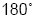d.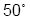b.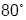e.c.3.

Measurement – Area, Perimeter, Circumference – RIT 211 – 220
Calculate the area of this shape.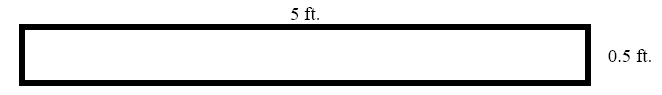a. 5.5 ft. d. 0.25 ft2 b. 2.5 ft2 e. 2.5 ft c. 25 ft2

4.

What happens to the perimeter when the length of a rectangle is
shortened?
 a. The perimeter stays the same. d. The perimeter is decreased by half. b. The perimeter becomes greater. e. The perimeter is increased by half. c. The perimeter becomes smaller.

5.

Measurement – Length, Weight, Volume – RIT 211 – 220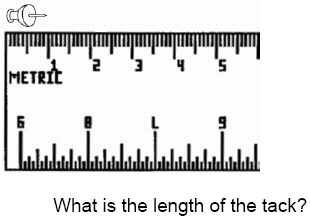a. 1 cm d. 1 m b. 2 cm e. 1 mm c. 5 cm

6.

Measurement – Money – RIT 211 – 220

If Jamaal will be paid \$5.00 an hour for delivering pizzas, and he works for
4 hours, how much will he be paid?
 a. \$9.00 d. \$2.00 b. \$5.00 e. \$20.00 c. 20

7.

Number Sense and Numeration – Decimals – RIT 191 – 200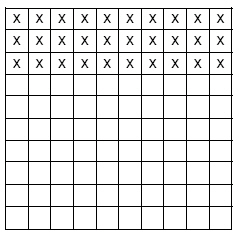What decimal does the shaded area represent?
 a. 0.33 d. .3 b. 0.03 e. 3.3 c. 1.3

8.

What is the value of the digit 4 in the number .246?
 a. 1/4 d. 4/100 b. 4/1 e. 100/4 c. 4/1000

9.

Number Sense and Numeration – Exponents – RIT 191 – 200

How is (5 x 5 x 5 x 5) represented in exponential form?
 a.d.b.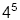e.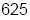c.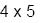10.

Number Sense and Numeration – Fractions – RIT 191 – 200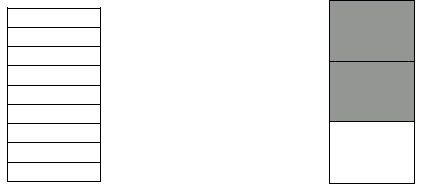=  2
9         3
 a. 7 d. 6 b. 4 e. 3 c. 8

11.

2/4 =___ /28
 a. 12 d. 14 b. 6 e. 32 c. 7

12.

Change 38/6 to a mixed number in simplest form.
 a. 6 2/6 d. 3 6/8 b. 6/38 e. 6 c. 6 1/3

13.

What is the LCD (least common denominator) of 4 and 3?
 a. 12 d. 8 b. 7 e. 18 c. 11

14.

1/5 expressed as a decimal =
 a. 0.25 d. .05 b. 0.2 e. .15 c. 0.5

15.

Which fractions are equal ratios? (Use the cross product method)
 a. 5/6, 9/12 d. 5/10, 7/14 b. 7/21, 6/9 e. 5/7, 10/15 c. 4/3, 9/16

16.

Number Sense and Numeration – Ordering, Equalities, and Inequalities –
RIT 191 – 200

Which expression is correct?
 a.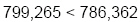d.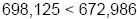b.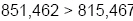e.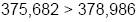c.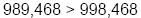17.

Which comparison is true?
 a.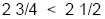d.b.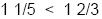e.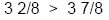c.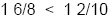18.

Number Sense and Numeration – Percents – RIT 191 – 200What percent is shown by this 100 unit figure?
 a. 9.5% d. 85% b. 95% e. 8.5% c. 850%

19.

1/4 = ____
 a. 20% d. 24% b. 25% e. 33% c. 30%

20.

Number Sense and Numeration – Place Value – RIT 191 – 200

What is 32 written as an ordinal?
 a. 3 tens, 2 ones d. thirty-two b. three-twos e. thirty-second c. thirtieth

21.

Put in expanded form: 406,219
 a. 400,000 + 60,000 + 200 + 10 + 9 d. 4,000 + 6,000 + 200 + 10 + 9 b. 46,000 + 200 + 10 + 9 e. 400,000 + 6,000 + 200 + 10 + 9 c. 40,000 + 6,000 + 200 + 10 + 9

22.

What number is named by four hundred thirty million, sixty-two thousand, four
hundred seven?
 a. 430,620,470 d. 462,407 b. 430,062,407 e. 430,602,407 c. 400,030,062,470

23.

The diagram below represents what number?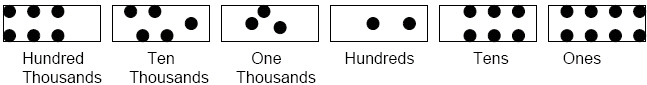a. 65,328 d. 563,268 b. 663,268 e. 635,628 c. 653,268

24.

Add and express in standard form.a. 117,630 d. 1,176 b. 1,763 e. 1,523 c. 11,763